# What is a Parallelogram?

A parallelogram is a quadrilateral in which both pairs of opposite sides are parallel. Special relationships exist between the measures of consecutive angles, opposite angles and opposite sides of a parallelogram. A square is the most straightforward parallelogram, because it has 2 sets of parallel sides. Naturally, all of its angles and sides match in length or measure.

(1) The degree measure of the four angles of a parallelogram add up to 360 degrees. Remember that all quadrilaterals (4 sided figures) have angles which add up to 360 degrees. Here's a sample: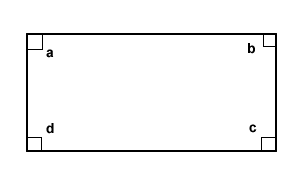Then: a + b + c + d = 360 degrees

(2) The degree measure of any two consecutive angles add up to 180 degrees. In parallelogram ABCD: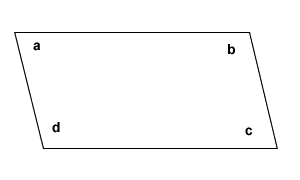angle a + angle b = 180 degrees
angle b + angle c = 180 degrees
angle c + angle d = 180 degrees
angle a + angle d = 180 degrees

(3) Opposite angles have the same measure in terms of degrees.

In parallelogram ABCD: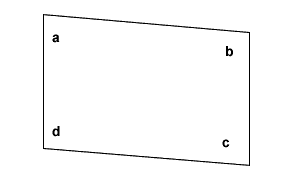angle a = angle c
angle b = angle d

### Sides of a Parallelogram

In parallelogram ABCD:

(1) Opposite sides are parallel: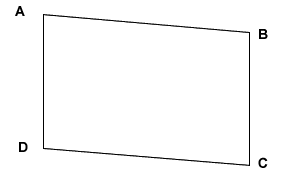side AB || side CD

NOTE: The symbol || means parallel.

(2) Opposite sides have the same lengths: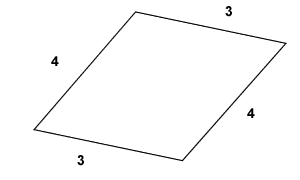side AB = side CD

### Diagonals of a Parallelogram

The diagonals of a parallelogram divide the parallelogram into two side-by-side triangles. As shown in the picture below, diagonal AC forms equal alternate interior angles with each pair of parallel sides. We can also see that there are two triangles in the picture below. Triangle 1 is congruent to triangle 2 by ASA (Angle-Side-Angle) Method.

Where did the two triangles come from? They were formed by diagonal AC.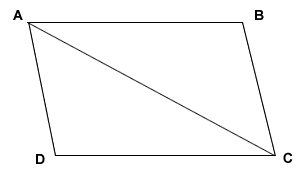I should also note that diagonals of a parallelogram bisect each other as shown in the picture below.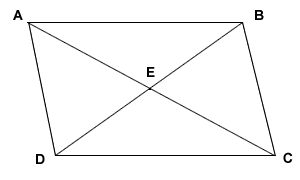AE = EC
DE = EB
where E is the midpoint of BOTH diagonals.

### Example:

In parallelogram WXYZ, the measure of angle X = 4a - 40 and the measure of angle Z = 2a - 8. Find the measure of angle W?

Solution:

(1) Find the value of a.

Since angles X and Z are opposite angles of parallelogram WXYZ, they have the same measure. We equate the terms and solve for a.

4a - 40 = 2a - 8
4a - 2a = 40 - 8
2a = 32
a = 32/2
a = 16

(2) Since a = 16, we now find the measure of angle X by substituting 16 for a in 4a - 40.

angle X = 4a - 40
angle X = 4(16) - 40
angle X = 64 - 40
angle X = 24

(3) We also know that consecutive angles of a parallelogram are supplementary (add up to 180 degrees) and so, this fact is used to find the measure of angle W.

angle W = 180 - angle X
angle W = 180 - 24
angle W = 156

Lesson provided by Mr. Feliz